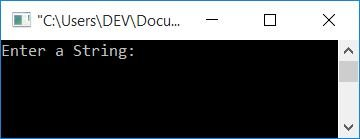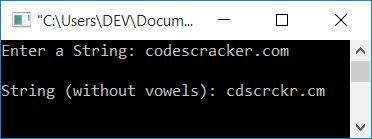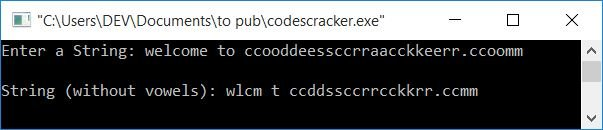# C Program to Delete Vowels from a String

In this article, you will learn and get code about how to delete or remove all the vowels from a given string. For example, if the given string is:

`codescracker.com`

Each of the 26 alphabetic characters has five vowels, as you are all aware. That are either A, E, I, O, and U (from the uppercase alphabet, A-Z) or a, e, i, o, and u (from the lowercase alphabet, a-z). Then, after deleting all the vowels from the given string, the string will become:

`cdscrckr.cm`

Now let's move on to the program.

## In C, remove vowels from a string

To delete vowels from the string in C programming, you have to ask the user to enter the string. Now check for a vowel (a, A, e, E, i, I, o, O, u, U). If any one gets found (of the 10), then move the next character to its one index back, until the last, and so on.

The question is, "Write a program in C that removes vowels from a given string by the user at run-time." The answer to this question is:

```#include<stdio.h>
#include<conio.h>
int main()
{
char str;
int i=0, j, chk;
printf("Enter a String: ");
gets(str);
while(str[i]!='\0')
{
chk=0;
if(str[i]=='a'||str[i]=='e'||str[i]=='i'||str[i]=='o'||str[i]=='u'||str[i]=='A'||str[i]=='E'||str[i]=='I'||str[i]=='O'||str[i]=='U')
{
j=i;
while(str[j-1]!='\0')
{
str[j] = str[j+1];
j++;
}
chk = 1;
}
if(chk==0)
i++;
}
printf("\nString (without vowels): %s", str);
getch();
return 0;
}```

You can also write the condition "if" block spreads into multiple lines, as shown below:

```if(str[i]=='a' || str[i]=='e' || str[i]=='i' ||
str[i]=='o' || str[i]=='u' || str[i]=='A' ||
str[i]=='E' || str[i]=='I' || str[i]=='O' ||
str[i]=='U')
{
j=i;
while(str[j-1]!='\0')
{
str[j] = str[j+1];
j++;
}
chk = 1;
}```

This program was built and runs in the Code::Blocks IDE. Here is the sample run:Now enter any string, such as codescracker.com, and press the ENTER key to see the output:Let's take another sample run in which the given string has more than one vowel present at each adjacent index.As you can see from the string,

`welcome to ccooddeessccrraacckkeerr.ccoomm`

have many times had vowels preset at the adjacent index. So the dry run using this input goes like this:

1. Variable str stores the string "welcome to ccooddeessccrraacckkeerr.ccoomm," in such a way that it can be parsed.
• str holds w.
• str holds e.
• str holds l.
• and so on.
2. The final character in str is m.
3. A null-terminated character "\0", on the other hand, is automatically initialized to str.
4. Initially, the value of i is 0.
5. At first run, the condition of the while loop evaluates to true because the character present at the 0th index (which is w) is not equal to a null-terminated character, so program flow goes inside the loop.
6. The value 0 is set to chk.
7. Because w is not a vowel, the first if condition evaluates to false.
8. As a result, program flow checks the condition of the second if block. There, it checks whether the value of chk is 0 or not.
9. Because it is 0, the condition evaluates to true, and the value of i gets incremented.
10. Now program flow goes back to the condition of the while loop and evaluates its condition to be true again because the character present at the 1st index (which is e) is not equal to a null-terminated character, so program flow goes inside the loop.
11. Process, 6th step
12. This time, because e is a vowel, the condition of the first if block inside the while loop evaluates to true.
13. The value of i gets initialized to j.
14. Shift all of the forward characters (from here) to the index one back.
15. That is, if the value of j is 1, then
• character present at index no.2 gets moved to index no.1.
• Similarly, the character at index no. 3 is moved to index no. 2.
• and so on.
• until a null terminated character occurs.
16. After shifting the character, now the current position of all the characters in the string variable str is such that
• str holds l.
• str holds c.
• str holds o.
• and so on.
17. From where the shifting gets performed, only the position of the character on the index gets replaced.
18. 1 gets initialized to the chk variable.
19. Here the initialization process is performed to check whether this loop is evaluated or not.
20. If evaluated, then don't increment the value of i.
21. Otherwise, increment the value of i.
22. And the program flow returns to the while loop's condition.
23. Because the variable's value is not incremented, it will be the same as the previous one, which is 1.
24. But this time, the character present in the first position is not e, but rather it is l.
25. Continue from step no. 7 with 'l' as a new character.
26. The process continues running until all the vowels are deleted from the string.

## Using a function, remove vowels from a string

This program uses a user-defined function called checkVowel() to check whether the current character is a vowel or not. If the character is a vowel, the function returns 1; otherwise, it returns 0.

To check for vowels, we simply used the function as the condition of an if block. Because 1 indicates a true condition and 0 indicates a false condition. Then, if function returns 1, then condition is evaluated to be true; otherwise, condition is evaluated to be false. Let's have a look at the program.

```#include<stdio.h>
#include<conio.h>
int checkVowel(char);
int main()
{
char str;
int i=0, j, chk;
printf("Enter a String: ");
gets(str);
while(str[i]!='\0')
{
chk=0;
if(checkVowel(str[i]))
{
j=i;
while(str[j-1]!='\0')
{
str[j] = str[j+1];
j++;
}
chk = 1;
}
if(chk==0)
i++;
}
printf("\nString (without vowels): %s", str);
getch();
return 0;
}
int checkVowel(char ch)
{
if(ch=='a' || ch=='e' || ch=='i' || ch=='o' || ch=='u' ||
ch=='A' || ch=='E' || ch=='I' || ch=='O' || ch=='U')
return 1;
else
return 0;

}```

This program produces the same output as the previous one.

#### The same program in different languages

C Quiz

« Previous Program Next Program »